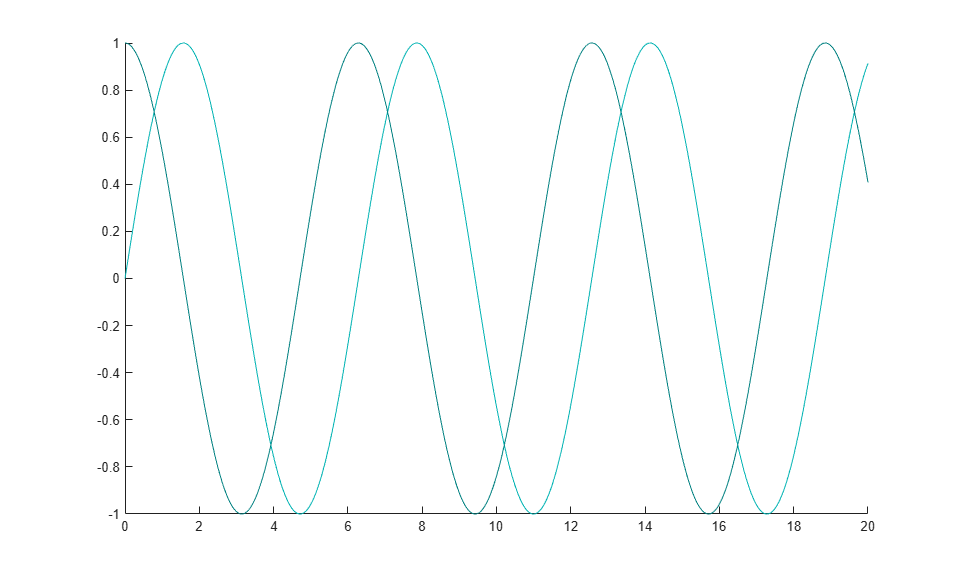# Line Animations

This example shows how to create an animation of two growing lines. The `animatedline` function helps you to optimize line animations. It allows you to add new points to a line without redefining existing points.

### Create Lines and Add Points

Create two animated lines of different colors. Then, add points to the lines in a loop. Set the axis limits before the loop so that to avoid recalculating the limits each time through the loop. Use a `drawnow` or `drawnow limitrate` command to display the updates on the screen after adding the new points.

```a1 = animatedline('Color',[0 .7 .7]); a2 = animatedline('Color',[0 .5 .5]); axis([0 20 -1 1]) x = linspace(0,20,10000); for k = 1:length(x); % first line xk = x(k); ysin = sin(xk); addpoints(a1,xk,ysin); % second line ycos = cos(xk); addpoints(a2,xk,ycos); % update screen drawnow limitrate end ```The animation shows two lines that grow as they accumulate data.

### Query Points of Line

Query the points of the first animated line.

```[x,y] = getpoints(a1); ```

`x` and `y` are vectors that contain the values defining the points of the sine wave.